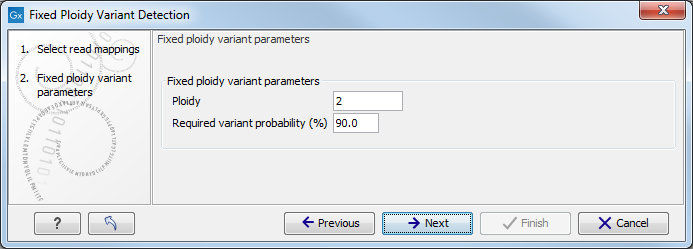# Fixed Ploidy Variant Detection

The Fixed Ploidy Variant Detection tool relies on two models:

1. A model for the possible 'site-types' depends on the user-specified ploidy parameter: For a diploid organism there are two alleles and thus the site types are A/A, A/C, A/G, A/T, A/-, C/C, and so on until -/-.
2. A model for the sequencing errors that specifies the probabilities of having a certain base in the read but calling a different base. The error model is estimated from the data prior to calling the variants (see The Error Model estimation).

The Fixed Ploidy algorithm will, given the estimated error model and the data observed in the site, calculate the probabilities of each of the site types. One of those site types is the site that is homozygous for the reference - that is, it stipulates that whatever differences are observed from the reference nucleotide in the reads is due to sequencing errors. The remaining site-types are those which stipulate that at least one of the alleles in the sample is different from the reference. The sum of the probabilities for these latter site types is the posterior probability that the sample contains at least one allele that differs from the reference at this site. We refer to this posterior probability as the 'variant probability'.

The Fixed Ploidy Variant Detection tool has two parameters: the 'Ploidy' and the 'Variant probability' parameters (figure 21.61):

• The 'ploidy' is the ploidy of the analyzed sample. The value that the user sets for this parameter determines the site types that are considered in the model. For more information about ploidy please see Ploidy and sensitivity.
• The 'Required variant probability' is the minimum probability value of the 'variant site' required for the variant to be called. Note that it is not the minimum value of the probability of the individual variant. For the Fixed Ploidy Variant detector, if a variant site - and not the variant itself - passes the variant probability threshold, then the variant with the highest probability at that site will be reported even if the probability of that particular variant might be less than the threshold. For example if the required variant probability is set to 0.9 then the individual probability of the variant called might be less than 0.9 as long as the probability of the entire variant site is greater than 0.9.Figure 21.61: The Fixed Ploidy Variant Detection parameters.

As the Fixed Ploidy Variant Detection tool strongly depends on the model assumed for the ploidy, the user should carefully consider the validity of the ploidy assumption that he makes for his sample. The tool allows ploidy values up to and including 4 (tetraploids). For higher ploidy values the number of possible site types is too large for estimation and computation to be feasible, and the user should use the Low Frequency or Basic Variant Detection Tool instead.

For a more in depth description of the Fixed Ploidy variant caller see Section 21.20.

Subsections